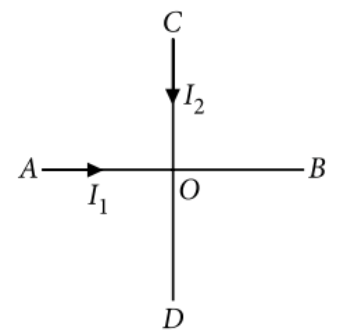Magnetic field due to a moving charge
Question

# Two identical long conducting wires AOB and COD are placed at right angle to each other, with one above other such that 0 is their common point for the two. The wires carry  currents, respectively. Point P is lying at distance d from 0 along a direction perpendicular to the plane containing the wires. The magnetic field at the point P will be

Easy
Solution

##The magnetic field at the point P, at a perpendicular distance d from 0 in a direction perpendicular to the plane ABCD due to currents through AOB and COD are perpendicular to each other. Hence, $B={\left({B}_{1}^{2}+{B}_{2}^{2}\right)}^{1/2}={\left[{\left(\frac{{\mu }_{0}}{4\pi }\frac{2{I}_{1}}{d}\right)}^{2}+\frac{{\mu }_{0}}{4\pi }{\left(\frac{2{I}_{2}}{d}\right)}^{2}\right]}^{1/2}=\frac{{\mu }_{0}}{2\pi d}{\left({I}_{1}^{2}+{I}_{2}^{2}\right)}^{1/2}$

Get Instant Solutions